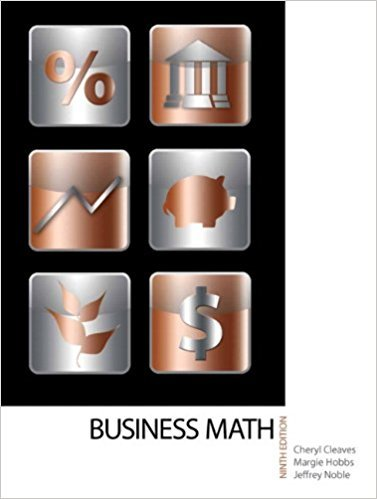×
×

# Solutions for Chapter 10-3: THE EMPLOYERS PAYROLL TAXES## Full solutions for Business Math, | 9th Edition

ISBN: 9780135108178Solutions for Chapter 10-3: THE EMPLOYERS PAYROLL TAXES

Solutions for Chapter 10-3
4 5 0 351 Reviews
27
2
##### ISBN: 9780135108178

This textbook survival guide was created for the textbook: Business Math, , edition: 9. This expansive textbook survival guide covers the following chapters and their solutions. Chapter 10-3: THE EMPLOYERS PAYROLL TAXES includes 7 full step-by-step solutions. Business Math, was written by and is associated to the ISBN: 9780135108178. Since 7 problems in chapter 10-3: THE EMPLOYERS PAYROLL TAXES have been answered, more than 17927 students have viewed full step-by-step solutions from this chapter.

Key Math Terms and definitions covered in this textbook
• Cayley-Hamilton Theorem.

peA) = det(A - AI) has peA) = zero matrix.

• Complex conjugate

z = a - ib for any complex number z = a + ib. Then zz = Iz12.

A sequence of steps (end of Chapter 9) to solve positive definite Ax = b by minimizing !x T Ax - x Tb over growing Krylov subspaces.

• Cramer's Rule for Ax = b.

B j has b replacing column j of A; x j = det B j I det A

• Cyclic shift

S. Permutation with S21 = 1, S32 = 1, ... , finally SIn = 1. Its eigenvalues are the nth roots e2lrik/n of 1; eigenvectors are columns of the Fourier matrix F.

• Four Fundamental Subspaces C (A), N (A), C (AT), N (AT).

Use AT for complex A.

• Fourier matrix F.

Entries Fjk = e21Cijk/n give orthogonal columns FT F = nI. Then y = Fe is the (inverse) Discrete Fourier Transform Y j = L cke21Cijk/n.

• Full column rank r = n.

Independent columns, N(A) = {O}, no free variables.

• Krylov subspace Kj(A, b).

The subspace spanned by b, Ab, ... , Aj-Ib. Numerical methods approximate A -I b by x j with residual b - Ax j in this subspace. A good basis for K j requires only multiplication by A at each step.

• Multiplication Ax

= Xl (column 1) + ... + xn(column n) = combination of columns.

• Network.

A directed graph that has constants Cl, ... , Cm associated with the edges.

• Orthogonal subspaces.

Every v in V is orthogonal to every w in W.

• Rotation matrix

R = [~ CS ] rotates the plane by () and R- 1 = RT rotates back by -(). Eigenvalues are eiO and e-iO , eigenvectors are (1, ±i). c, s = cos (), sin ().

• Schwarz inequality

Iv·wl < IIvll IIwll.Then IvTAwl2 < (vT Av)(wT Aw) for pos def A.

• Similar matrices A and B.

Every B = M-I AM has the same eigenvalues as A.

• Skew-symmetric matrix K.

The transpose is -K, since Kij = -Kji. Eigenvalues are pure imaginary, eigenvectors are orthogonal, eKt is an orthogonal matrix.

• Stiffness matrix

If x gives the movements of the nodes, K x gives the internal forces. K = ATe A where C has spring constants from Hooke's Law and Ax = stretching.

• Subspace S of V.

Any vector space inside V, including V and Z = {zero vector only}.

• Unitary matrix UH = U T = U-I.

Orthonormal columns (complex analog of Q).

• Vector v in Rn.

Sequence of n real numbers v = (VI, ... , Vn) = point in Rn.

×